## Wednesday, 29 December 2010

### The Poisson Variation of the Binomial

My recent post about Why the Other Line Moves Faster reminded me of another variation of the binomial distribution that is not covered in AP Statistics, the Poisson Distributions. (Ok, the Poisson is lots more than just a special case of the binomial, more about that later, but in the days before hand-held calculators it was in that sense that we met it.)

The distribution is named for the French Mathematician Simeon Denis Poisson (hey, his name is on the Eiffel Tower.. 2nd from the right on the South East side).Suppose you had a very rare binomial event, let's say something that happened only one in 200 trials (p=.005) and we wanted to know how probable it was to happen four times (x=4) in three-hundred trials (n=300). The calculation of the binomial probability is now as easy to do with modern calculators as with the Poisson approximation to it, but in my youth a calculation of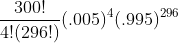looked nearly impossible.

The Poisson frequently uses the Greek letter lambda,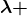, for the mean or expected value np. In this case NP=300(.005) = 3/2 indicates that, on average, we would expect only 1.5 successes in 300 trials. We want to calculate the probability of getting four successes. The Poisson probability is given by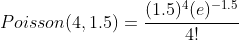. The difference between the two calculations is less than .0002 on my Ti-84 calculator.

The Poisson is not limited to binomial events. More often it is applied to events which are distributed randomly across time. The same calculation above could be used to calculate the probability of four people entering a bank in a 15 minute period when only and average of 1.5 visitors would be expected. If a manufacturing process produces an average of 1.5 failures per day and you wanted to calculate the probability of four failures, you use the same calculation again.

And if you wanted to know how many cashiers to keep open at the market, how many mutations in a string of DNA exposed to radiation, the number of deaths from a rare side effect of a drug, or other similar situations which involve a very rare event with a very large number of possible incidences, the Poisson will often suffice as long as conditions of independence are met.

The Poisson distribution is sometimes called the Law of Small Numbers after a book by the same name by Ladislaus Bortkiewicz published in 1898 on the Poisson distribution.

For AP Statistics Students, it is often possible to approximate the Poisson with the Normal Distribution as long as the value of lambda is about ten or more. For the Poisson, the mean and variance are both the same, so if you approximate it with a normal, use a mean of lambda, and a standard deviation that is the square root of lambda. Do remember to do the continuity correction for the fact that the Poisson is discrete and the Normal is continuous.

### Why the Other Line is More Likely to Move Faster

One of my ex-students, Chad Brinkman, sent me a link to this video by Bill Hammack, "The Engineer Guy,". A nice view for any math student.

Video: Why the other line is more likely to move faster## Friday, 24 December 2010

### A Happy Holiday to All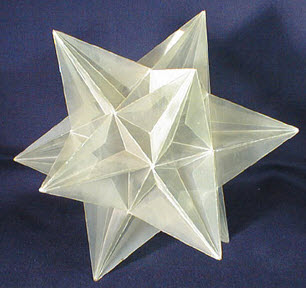Until the world finds the peace we dream of... May you find peace in your own heart.

And a carol I snitched from John D. Cook at his "Endeavour" Blog

## Thursday, 23 December 2010

### More "Almost Binomial" Distributions

In my recent post I illustrated the extension of the binomial to a Multinomial Distribution. In a similar way, the geometric distribution and the Pascal (aka the Negative Binomial) Distribution are very much like special cases of the binomial.

I will illustrate each with a simple probability example. In a limited version of the game of "greedy pig" you roll a die as many times as you wish each turn and you add the points on the top of the die to your score for that turn...but... if you roll a one, your turn ends and you lose all the points you have earned for that round. One might inquire, what is the probability that you could roll the die n times without rolling a one. Since the probability on each roll is the same, we could handle this using the binomial (or multinomial) distribution with n trials, p=5/6, and the number of successes also equal to n. For n=5 for example, we get (using the notation established in that blog)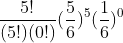which simplifies to just (5/6)5 .

But a slightly different question might be, what is the probability that our first failure (rolling a one) would occur on the sixth roll. This is asking for the probability that the first five rolls succeed, and then the final roll is a failure. This is the general model for a geometric distribution. The reason it is called a geometric distribution is clear if you calculate the probability of the first failure happening on the first, second, etc rolls.

Roll...1....2.......3........4...

Prob...1/6..5/36...25/216 ..5^3/6^4..

notice that each probability is the previous probability multiplied by a constant ratio of 5/6. The terms for a geometric sequence (which must sum to one to be a probability distribution......check)

In general, if the probability of failure is q = 1-p.. then the probability of the first failure occurring on the nth trial is given by (p)n-1(q)

It often surprises students that the mean for such a distribution is 1/q where q is the probability of a failure. OK before I confuse someone.. the geometric distribution is sometimes described as the number of trials to the first success, so you may see the expected or mean value as 1/p. In any event, if the probability of an event happening (whether you call it success or failure) is p, the expected number of trials before it happens is 1/p.

Now if you are really clever you can figure out how to do the next problem without me, but let's walk through it anyway, (hey...it's MY blog).
Suppose instead, you could keep rolling until you had three rolls of one..... sort of "three strikes and you're out." Now what is the probability that the third strike comes on the tenth roll.
The idea of course, a collection of 9 rolls with 2 failures anywhere in the string, and then a third failure on the tenth roll. To get the probability of all the possible ways to get 7 successes and 2 failures in the first nine rolls is a straight binomial (multinomial) probability problem.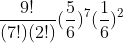We just multiply this by a failure on the tenth roll and we have the probability we seek. Since we have a couple of "failures" in that (1/6)^2, we might as well just up it to a three and be done. The final probability is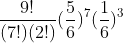If you would like to experiment with these distributions, I came across a nice experimental applet hereThis experiment uses the trials to k successes instead of failures, and so p and q are switched here (and it seems I could only adjust these in .05 increments). This is a nice routine and you can simulate trials by clicking on the "step" button to see how many trials it took to get three successes.
This applet is part of a nice virtual laboratory created by Kyle Siegrist of the Department of Mathematical Sciences at the University of Alabama in Huntsville. There is lots of nice stuff. See the home page here.

When we deal with integer numbers of failures this is called a Pascal Distribution after Blaise Pascal. It can be extended to any real and is then called a Polya Distribution, after George Polya. This has application for events which are very rare, but related to each other, such as hurricanes. Both are special cases of the general Negative Binomial Distribution.

## Wednesday, 22 December 2010

### Extending the Binomial Distribution

Almost every high school student is exposed to the binomial distribution in some form. They may see it in expanding binomials such as (x+y)4, and they may also come across it as a method of solving simple probability problems... "what is the probability that a family with four children will have three boys and one girl?"

The ability to naturally extend the binomial (or to recognize that the two questions above are interrelated) is probably hampered by the notation used in the "choose" command, or the various combination notations. The normal way for students to address the problems about combinations is to think of one group embedded in the total field. They may useto find three boys, or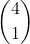to find one girl. Both methods lead to the same calculation,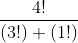but they seem to direct the focus of the learner away from the idea of "three of these and one of those" which would embed the problem firmly in the multinomial distribution. I suspect that if the "choose" or "combination" notation was not used, many students would almost naturally extend the binomial probability problem to similar problems with three (or more) item choices.

For students who have never seen the multinomial I will provide a brief introduction, and a few good links.

Suppose instead of two choices to pick from, a population had three choices..(the extension to four should jump out at you). A spinner has the numbers one, two, and three on it with probabilities of 1/6, 1/3, and 1/2 respectively. What is the probability that in ten spins you would get 2 ones, 3 twos, and five threes. The probability is simply given by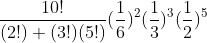The association between the number of things selected in all (10) and the number and probability of the individual partition seems to be naturally extendable to any number of items. Keep in mind that, like the binomial, this requires that the probability on each draw is unchanged... we are drawing with replacement or from an "infinite" pool. This does require that the sum of all the probabities add up to one

You can use this to extend the expansion of a binomial to the expansion of any polynomial to a power. To make this clear to new learners, I will go back to the idea that (x+y)4 is related the probability of three boys and one girl in a family of four children. To do that, I want to give a verbal expansion of (x+y)4, but instead of x and y I will use b and g for (boys probability of birth and girls probability of birth... well, they might not be 1/2). The expansion of (b+g)4 will give the probability of every possible outcome, 4 boys and no girls, 3 boys and one girl, two boys and two girls, one boy and three girls, and no boys and four girls. Each term in the expansion represents one of these cases. For four boys and no girls, we have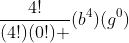, this is added to each succeeding term until we end with no boys and four girls. This gives exactly the expansion you would have for (x+y)4 except for the use of b and g as variables.

To extend this to a trinomial we get a few more terms, but we can just attack them systematically as there is no such natural approach as there is in the binomial case. For instance, if we had (a+b+c)2 we could have two a's, two b's, two c's, or ab, ac, or bc so there must be six terms. It may help to think of it as (a+b+c)(a+b+c) and you pick one term from the first trinomial and one from the second to multiply. The three squared terms will have coefficients of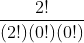which is a big one. The ones where we pick two different ones will be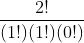, which can occur two ways. So we get a2+b2+c2+2ab+2ac+2bc.

If you want to try (a+b+c)3 then you will get ten terms. In fact for any power n, a trinomial will have the n+1st triangular number which coincidentally is (n+1) choose 2. There is even an extension of Pascal's triangle, called Pascal's tetrahedron that can be used but you have to create it (or at least I do) level by level. I find it usually easier to just do the multinomial coefficients. You can find a pretty good explanation of the tetrahedron here. There is also a good wikipedia page about the multinomial distribution.

## Tuesday, 21 December 2010

### Cool Doodles

One of my favorite ex-students sent me a link to this video by Vi Hart. I loved it, but I hope Jessica didn't find my math class as awful as Vi describes them. Anyway, enjoy.

Vi has her own web page with lots of math and music stuff.

## Saturday, 18 December 2010

### "Decimal" Fractions in Other Bases

Early in my study of decimal fractions I realized that the ninths were just repeating digits of their numerators. 1/9 = .111...; 5/9 = .5555... etc. I didn't have much to apply it to, but it sort of fascinated me. Somewhere in the sixth grade or so, we were introduced to bases other than ten. Something about Sputnik made American education decide that base two and base five was important. I was fascinated again, but when I became curious about "decimal" fractions in other bases, my teacher advised me that, "We don't cover that." {If you know a good name for the general term of such fractional expansions, please advise.} Thanks to GasStationWithoutPumps, I now know this is called a radix expansion. Radix is from the same base that gives us "root".

Later I began to understand polynomials better, and realized that I could extend base n whole numbers across the "decimal" point as far as I wanted using the idea I would now describe as negative powers (not sure I had a word for it then). Armed with the idea that .1 in base 2, or .1  was 1/2, and .1 in base 5, .1 was 1/5 I began trying to construct sets of fractions. Moving the "decimal point" one to the left in base two divided the result by two in the same way that it divided by ten in base ten. With that, I could produce most of the fractions that terminated, .0101 was 1/4 plus 1/16 or 5/16, .23 was 13/25 (2*5+3)/52.

Then I read about the formula for infinite geometric series with ratios less than one. I think the article was about Archimedes use of the series, but I couldn't understand the center of gravity approach at that time. What I did realize was that I could use the formula to convert any non-terminating decimal fraction to a rational fraction..... King of the World. I would write out strange fractions that had non-repeating prefixes to the repeat. Soon I began to wonder about repeating decimals in other bases and set out to explore. Remembering the repunit expansion for 1/9, I wondered about .1111... in other bases. I was kind of shocked to realize that .11111.. was =1. How could that happen? But I had already read about "proofs" that .99999.. = 1; and quickly convinced myself that in base n, a repetend of the digit (n-1) would also be one. But somewhere along the way, I realized that .1111...[n] would be 1/n-1. Just as it was equal to 1/9 in base ten, it was 1/4 in base five, or 1/2 in base three.

With all this experience, I still found it very hard to pick a random fraction, say 4/7, and express it in base 3 or base 5 or whatever I wished. Then one day I learned about division. Ok, I had learned long division and short division and mental division tricks, but I didn't really know how division worked. I'm not sure what I was reading, thumbing through books in the public library, and the author showed a shortcut for making "decimal" fractions in base two. What seemed like a magic trick became understanding when I began to extend it to other bases.

To understand, I want to do a simple division in base ten written a little differently than you normally would. For an example I will use 1/8. Set up the operation in four columnsSince 8 will not divide into 1, we have a fractional answer and we will multiply one by ten and try again (this is actually dividing the number of tenths by eight. This time 8 will go into ten once, with a remainder of two. This is shown in the second line.The remainder is 2 multiplied by 10 (to get the 20) and we divide by eight again. This continues until we either terminate, or enter a repeating pattern. Here is the final table giving us the expected .125 for an answer.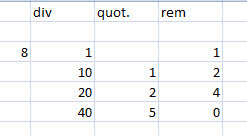The question is what is special about ten, and the big answer is ..... nothing. We could divide the fraction in any base by simply using some other multiple in place of the ten in each line. Here is 1/3 in base five.Notice that the occurrence of the remainder of one means we will repeat the same sequence forever, so our answer is .13131313...  = 1/3. We can convince ourselves this is correct by using the geometric series. The first two digits are 1(5)+3=8 and represent 8/25. The next repetition of 13 is 8/252 , and each two digits in the sequence is 1/25 of the previous two. This is a geometric sequence with a first term of 8/25, and a common ratio of 1/25. Using the well known formula for such series gives 8/24 = 1/3..

Ok, one more example of the division method to help you.... this time we pick base three, and let's try to represent 2/5 in that base.2/5 = .101210121012..... OK, one more quick tip. Most students know that any repeating decimal fraction can be written as a rational by just subtracting one from the denominator of the repetend (say WHAT?) ok.. .4 repeating is 4/9 (four tenths repeating); and .232323... is 23/99. It doesn't matter how long the repeat cycle is, as long as it starts right from the decimal point; .12345 is just 12345/99999..... and you can do that in ANY base...
so a fraction like .1012 in base three can be written as its base three fraction and then apply the same rule. 1012 in base three is 1(27)+0(9) + 1(3)+2(1) so the numerator is 32 in base ten, and the denominator is 34 or 81. The rule for repeating is subtract one from the denominator, so .10121012... is 32/80 = 2/5....

.101 repeating in  = 5/(23-1)= 5/7
.101 repeating in  = 10/(33-1)= 10/26= 5/13
.31 repeating in = (3(4)+1)/(42-1)= 13/15
.31 repeating in = 16/(52-1)= 16/24 = 2/3
.31 repeating in = 19/(62-1)= 19/35

Fun with fractions!!!

## Wednesday, 15 December 2010

### Viete on Combining Pythagorean Triples

Not able to write much this holiday season, but here is one I really enjoy from a few years ago.. hope it is of interest...

Reading The Analytic Art by Francois Viete, or at least the T R Witmer
translation, and came across an interesting way of combining the legs of any two Pythagorean triples to create two others. Viete calls the two methods synaeresis and diaeresis, which seem to be language terms Viete appropriated. Synaeresis is cramming two vowel sounds together to make one... like the way people in New Orleans say "Nor"leans. I think the official term is diphthong, but check with an English major for confirmation. The actual Greek roots mean “a joining or bringing together" or something similar Diaeresis is stretching one vowel out into two....and you can find your own example...
To illustrate Viete's approach, we can take two simple right triangles, say a 3-4-5 and a 5-12-13 as examples. Viete's method would produce two triangles whose hypotenuses( hypotenii?) were both 5x13 = 65 units. Viete distinguished between the legs calling them base and the perpendicular, so in the 3-4-5 triangle the base is 3 and the perpendicular is 4. It doesn't matter which is called what name, of course except that it reverses the outcomes of the two methods. The Synaeresic method would be to add the products of each base with the perpendicular of the other triangle; 3x12+ 4x5 = 56. This would give one leg of the new triangle. To find the other leg take the difference of the products of the two bases from the two perpendiculars; 4x12 - 3x5 = 33. This completes a triple of 33-56-65.
The second method, simply reverses the signs of conjunction. Subtract the two perpendicular x base products and add the two products of a common part. The crossed terms gives 3x12-4x5 = 16 for one leg, while the products of like parts gives 4x12+3x5=63 for the other, completing a 16-63-65 right triangle.